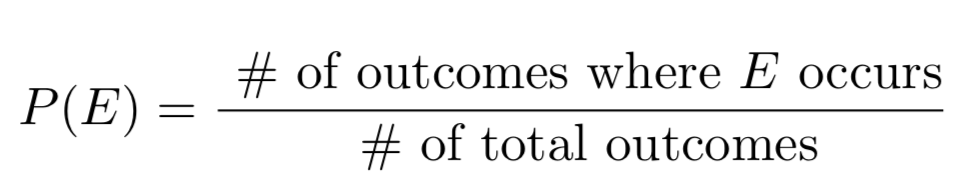In our previous posts, we've talked about the basic concepts of probability and some fundamental facts about probabilities. Here, we'll show how to calculate the probability of a single event when all the outcome are equally likely. This is, in a sense, the simplest case that we will cover, and it is crucial for everything we'll do later (e.g. in finding the probability of two events occurring).

Suppose we flip a fair coin. What is the probability we get heads? Intuitively, the answer should be 1/2. And that's exactly what the following rule would say:

Probability of Equally Likely Outcomes:
If you have n possible outcomes, all of which are equally likely, then the probability of any particular outcome occurring is 1/n.

So when we flip a fair coin, there are 2 possible outcomes (heads and tails). So n = 2 and the probability of one outcome (e.g. heads) occurring is 1/n = 1/2. And if we roll a six-sided die, there are 6 possible outcomes. So the probability of any particular outcome (e.g. rolling a 4) is 1/6. And if we held a raffle where there were 109 different entrants, the probability of any one of them winning would be 1/109.

Note that this rule only applies when all the outcomes are equally likely. In most GRE problems, the outcomes will be equally likely, and the question will signal that by saying that the outcome is "random" or that the outcomes are "equally likely." So, the question might say things like: "a name is chosen at random" or that "each outcome is equally likely." When the outcomes are not equally likely, all bets are off, and you will have to be more careful in how you approach the problem.

Now, we want to find the probability of some event occurring. Suppose I am going to roll a six-sided die, numbered 1 through 6. What is the probability that I get an even number? To calculate this, we use the following rule:

Probability of Single Events (for equally likely outcomes)
Suppose you have n equally likely outcomes. Then, the probability of some event E occurring is:where the # of total outcomes = n.

So to find the probability of rolling an even number, we need to find the number of outcomes where we roll an even number. If we roll an even number, then we must have rolled a 2, 4, or 6. Then, we divide by the number of total outcomes, in our case 6. So, P(Roll an even number) = 3/6 = 1/2.

Here’s another example in a similar spirit:

Example 1
Suppose you randomly choose a number from 1 to 50. What is the probability that you chose a prime number?

Now we know how to find the probability of a single event when the possible outcomes are equally likely. Our next step is to learn how to combine these probabilities in order to get the probabilities of more complex events.

Practice Problems

Question 1
130 people line up to buy raffle tickets. Every 10th person who buys a ticket gets a teddy bear as a promotional item. What is the probability that a randomly chosen person from the line will receive a teddy bear?

Question 2
You have 50 friends. 12 of them have blue hair. You randomly pick one of your friends to invite to dinner tomorrow. What is the probability that you invite a person with blue hair?

Question 3
You still have 50 friends. 12 of them still have blue hair. What is the probability that you do not invite a person with blue hair?

Next Article: Probability for Two Events to Both Occur - P(A and B)